# Triangular Cross Section Civil Engineering (CE) Notes | EduRev

## Civil Engineering (CE) : Triangular Cross Section Civil Engineering (CE) Notes | EduRev

The document Triangular Cross Section Civil Engineering (CE) Notes | EduRev is a part of the Civil Engineering (CE) Course Advanced Solid Mechanics - Notes, Videos, MCQs & PPTs.
All you need of Civil Engineering (CE) at this link: Civil Engineering (CE)

Triangular cross section

Finally, we consider the torsion of a cylinder with equilateral triangle cross section, as shown in figure 9.21. The boundary of this section is defined by,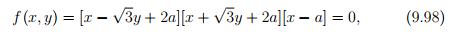where a is a constant and we have simply used the product form of each boundary line equation. Assuming that the Prandtl stress function to be of the form,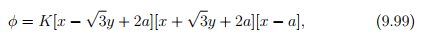so that the boundary condition (9.50) is satisfied. It can then be verified that the potential given in equation (9.99) satisfies (9.47) if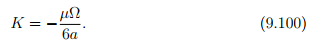Substituting equation (9.99) in (9.57) and using (9.100) we obtain the torque to be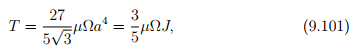where the polar moment of inertia for the equilateral triangle section, J = 3 √ 3a4 .

The shear stresses given in equation (9.44) evaluates to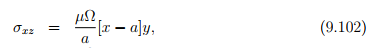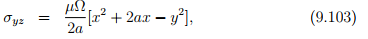on using equations (9.99) and (9.100). The magnitude of the shear stress at any point is given by,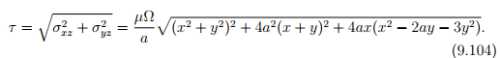Since, for torsion the maximum shear stress occurs at the boundary of the cross section, we investigate the same at the three boundary lines. We begin with the boundary x = a. It is evident from (9.102) that on this boundary σxz = 0. Then, it follows from (9.103) that σyz is maximum at y = 0 and this maximum value is 3aµΩ/2. It can be seen that on the other two boundaries too the maximum shear stress, τmax = 3aµΩ/2. Substituting (9.99) in equations (9.45) and (9.46) and solving the first order differential equations, we obtain the warping displacement as,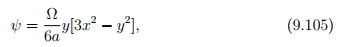on using the condition that the origin of the coordinate system does not get displaced; a requirement to prevent the body from displacing as a rigid body.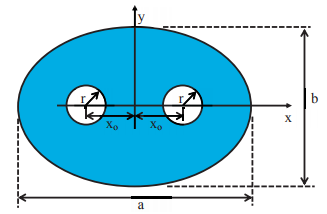Figure 9.22: Example of a multiply connected cross section. Elliptical cross section with two circular holes.

Offer running on EduRev: Apply code STAYHOME200 to get INR 200 off on our premium plan EduRev Infinity!

## Advanced Solid Mechanics - Notes, Videos, MCQs & PPTs

42 videos|61 docs

,

,

,

,

,

,

,

,

,

,

,

,

,

,

,

,

,

,

,

,

,

;